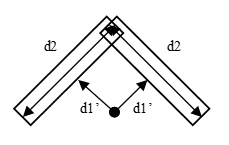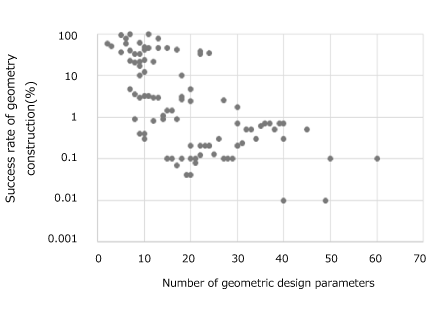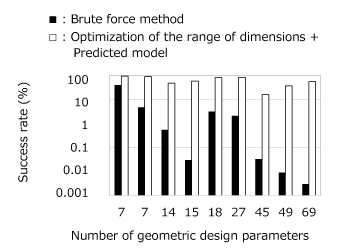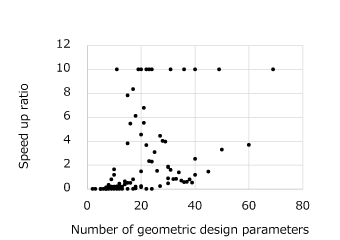## [W-OP-165] Solving Geometry Conflicts in GA Optimizations with Large Numbers of Geometric Parameters

Contents
1. Introduction
2. Proposed method
2.1 Range Optimization
2.2 Predictive model
2.3 Performance
3. Application on IPM with 30 geometric parameters
4. Summary

### 1. Introduction

Genetic Algorithm (GA) based optimization with finite element analysis is expected to become a solution for the high performance motor designs which need to satisfy many strict requirements. However, as its use goes towards complex design which having many geometric design parameters, the technique becomes difficult to use. The problem comes from the fact that the geometric design parameters move independently in given ranges so that there is always the possibility of them conflicting with each other and resulting in a geometries collapse as illustrated in Fig 1. The possibility goes up rapidly as the number of the geometric design parameter increases as the survey of the success rate of multiple models demonstrates in Fig 2. The geometry conflicts then prevent generating an appropriate population at the initial stage of the GA optimization. To overcome this, a technique which consists of an optimization of parameter ranges and a predicted model using machine learning was developed. The proposed technique was applied to an optimization problem of a PMSM with over 30 geometric design parameters confirming that the initial population was appropriately generated and the optimization can be performed successfully.Fig. 1 Geometry Conflict
Two geometric design parameters d1’ and d2 are specified separately. When d1’ is short and d2 is large, two rectangle regions collapse. To avoid the conflict, it requires to set d1’ and d2 appropriately. When the number of geometric design parameters is large, the setting all parameters becomes difficult.Fig. 2 Success rates of geometry construction versus the number of the geometric design parameters
When the number of geometric design parameters is larger than 30, the success ratio of geometry construction by the brute force method which generating individuals ignoring conflicts until the number of the individuals reaches the required initial population size is less than 1%.

### 2. Proposed method

The proposed method is separated into two parts, the first is the range optimization to update the ranges of the geometric parameters and a predictive model used to create the valid cases. The second is the use of the predictive model to obtain the required number of individuals.

### 2.1 Range Optimization

The range optimization uses a GA algorithm that tries to maximize the ranges of the geometric parameters while minimizing the geometric conflicts. This will result in ranges that will provided a higher success rate without drastically reducing the design space.

### 2.2 Predictive model

For further improvement, a machine learning based predicted model is introduced. The predicted model outputs whether is a conflict in the geometry for a given set of geometric design parameters. Using this the selection of only success cases can be performed in shorter time than constructing actual geometry models. Although the accuracy of the predicted model depends on the amount of the learning cases each of which requires an actual geometry model construction, the total generation time using the predicted model including the learning time is shorter than the brute force approach.

### 2.3 Performance

The proposed technique will provide a better success rate in the creation of the first generation for the GA optimization. The improvement provided by this method increases with the number of geometric parameters shown in Fig. 3. The proposed technique maintains high success rate and greatly reduces the generation time for the initial population. The effect becomes significant as the number of the parameters increases as shown in Fig. 4.Fig. 3 Success rate of geometry construction by different combination of methodsFig. 4 Speed up ratio by using the range optimization and the prediction model
The reference is the time by using the brute force approach. Points that the ratio is more than 10 are plotted on the line of 10 times.

Protected content here, for members only.
You need to sign in as a JMAG software regular user (paid membership) or JMAG WEB MEMBER (free membership).

By registering as a JMAG WEB MEMBER, you can browse technical materials and other member-only contents for free.
If you are not registered, click the “Create an Account” button.

Remember me